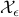# Techniques for exploring the suboptimal set

J. Skaf and S. Boyd

Optimization and Engineering, 11(2):319-337, June 2010.

The-suboptimal setfor an optimization problem is the set of feasible points with objective value withinof optimal. In this paper we describe some basic techniques for quantitatively characterizing, for a given value of, when the original problem is convex, by solving a modest number of related convex optimization problems. We give methods for computing the bounding box of, estimating its diameter, and forming ellipsoidal approximations.

Quantitative knowledge ofcan be very useful in applications. In a design problem, where the objective function is some cost, largeis good: It means that there are many designs with nearly minimum cost, and we can use this design freedom to improve a secondary objective. In an estimation problem, where the objective function is some measure of plausibility, largeis bad: It means that quite different parameter values are almost as plausible as the most plausible parameter value.